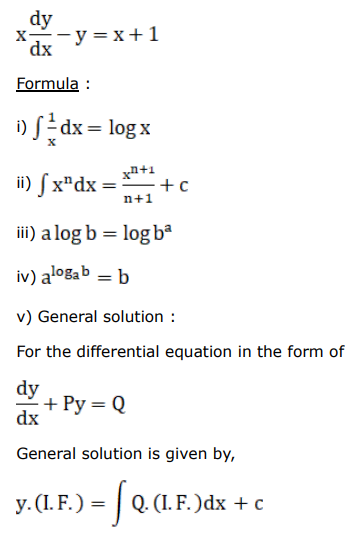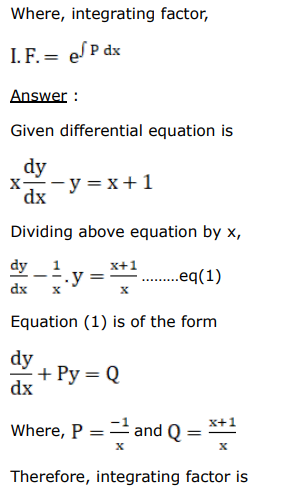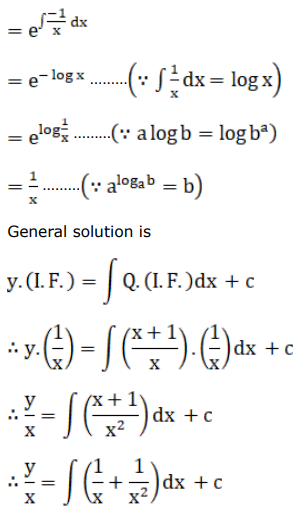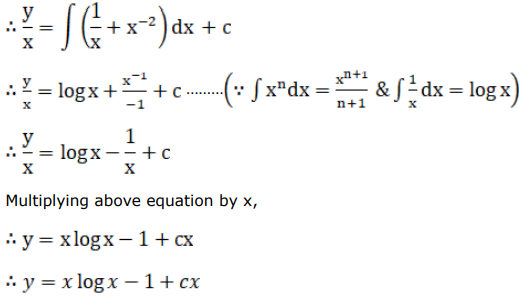# Find the general solution for each of the following differential equations.`
Question:

Find the general solution for each of the following differential equations.

$x \frac{d y}{d x}-y=x+1$

Solution:

$\underline{\text { Given Differential Equation }}$ :I. F. $=e^{\int P d x}$